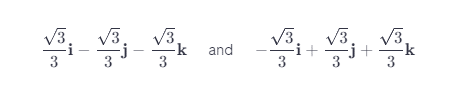# Find A Unit Vector That Is Orthogonal To Both I + J And I + K.

We thoroughly check each answer to a question to provide you with the most correct answers. Found a mistake? Let us know about it through the REPORT button at the bottom of the page.

Find a unit vector that is orthogonal to both i + j and i + k.

Contents

The cross product will produce a vector orthogonal to both.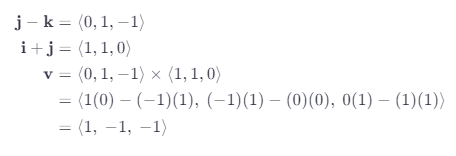Find the length of v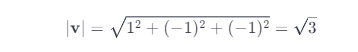Divide the components of v by this to make it have a unit length (normalize it)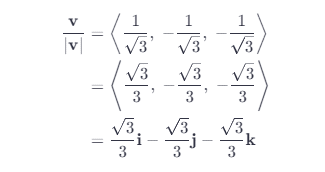To get the other orthogonal vector we can just negate this to become: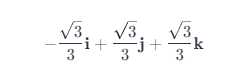## Result